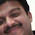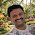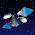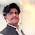## Saturday, July 23, 2011

### Lens Thickness Formula !

After reading my last post for the basic thickness calculator, I have received some mails were asking me for the formula to find the thickness. (In that post, the formula was hided from the users)

Here I am explaining how to find the estimated thickness of a lens before it makes. To find the thickness you need to know the power, index, diameter and the edge/ center thickness.

For plus power you have to consider the edge thickness and for the minus powers you have to consider center thickness. (usual range is 0.9mm to 2.00mm according to the index)

Formula:
Thickness= [(1/2*Diameter)^2*Power / [2000*(Index - 1)]] + edge/center thickness

For instance, given a lens power of -3.75 D, a refractive index of 1.53, and a minimum blank size of 65 mm, with 1.50mm center thickness, then the thickness would be:

[(1/2*65)^2*3.75 / [2000*(1.53 - 1)]] + 1.50 = 5.24 mm

For the ease of use I have created an excel file, there you can enter all the input values for the result (fig. 1).

1.HI, i still got one question: Your formula calculate thickness for a spheric lenses. How do i calculate thickness for the aspheric lenses?

2.Power +0,25
Diameter 65
Index 1,5
Edge thickness 0,5mm

The calculated center thickness is 0,76mm.
You will not get this lens from any supplier.

I would like to have ASPH formulas as well.

3.@Anonymous

The formula is correct and verified by us. But unfortunately the edge thickness you have mentioned is too low. For this type of small powers, stock lenses are coming with 1+ edge thickness.

4.Been trying to download the lens thickness finder, but without succes. Does anybody have a copy.

regards

Jens

5.Pls repost the download link of both the lens thickness calculator in excel & basic thickness calculator

regds

6.how do i work out the power of a lens when i only have the diameter and thickness?

1.that will be quite difficult. It is possible only with non aspherical lenses and need edge, center thicknesses along with its diameter.

7.8.Why I have problems with the bevel lens in the mei edge and with the lens dimension.

1.Which MEI machine are you using?

9.Thickness= [(1/2*Diameter)^2*Power / [2000*(Index - 1)]] + edge/center thickness
i do not known where is 2000

10.Pls let me known. tks so much

11.how to caculation with ED IN PROGRESSIVE LENS. i ccan not understain because it with ADD

12.this is wrong farmoula